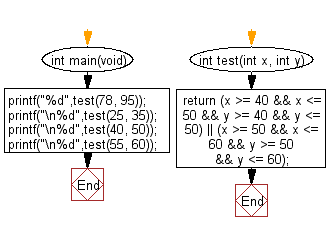﻿ C : Compare two integers in the range 40..50 or 50..60# C Exercises: Check whether two given integers are in the range 40..50 inclusive, or they are both in the range 50..60 inclusive

## C-programming basic algorithm: Exercise-11 with Solution

Write a C program to check whether two given integers are in the range 40..50 inclusive, or they are both in the range 50..60 inclusive.

C Code:

``````#include <stdio.h>
#include <stdlib.h>
int main(void){
printf("%d",test(78, 95));
printf("\n%d",test(25, 35));
printf("\n%d",test(40, 50));
printf("\n%d",test(55, 60));
}

int test(int x, int y)
{
return (x >= 40 && x <= 50 && y >= 40 && y <= 50) || (x >= 50 && x <= 60 && y >= 50 && y <= 60);

}
``````

Sample Output:

```0
0
1
1
```

Pictorial Presentation:Flowchart:C Programming Code Editor:

What is the difficulty level of this exercise?

Test your Programming skills with w3resource's quiz.

﻿

## C Programming: Tips of the Day

Why doesn't a+++++b work?

printf("%d",a+++++b); is interpreted as (a++)++ + b according to the Maximal Munch Rule!.

++ (postfix) doesn't evaluate to an lvalue but it requires its operand to be an lvalue.

! 6.4/4 says the next preprocessing token is the longest sequence of characters that could constitute a preprocessing token"

Ref : https://bit.ly/3fdldUT